### Home > MC2 > Chapter 2 > Lesson 2.3.1 > Problem2-98

2-98.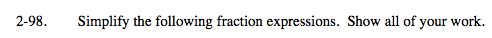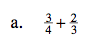Draw diagrams to represent each fraction.

Now change the diagram to have common denominators. Then solve.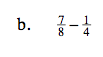Draw a diagram like part (a) to help you solve this problem.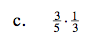Draw a rectangle divided in to thirds and fifths!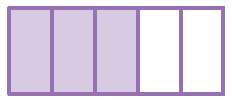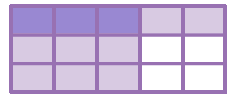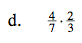$\frac{8}{21}$